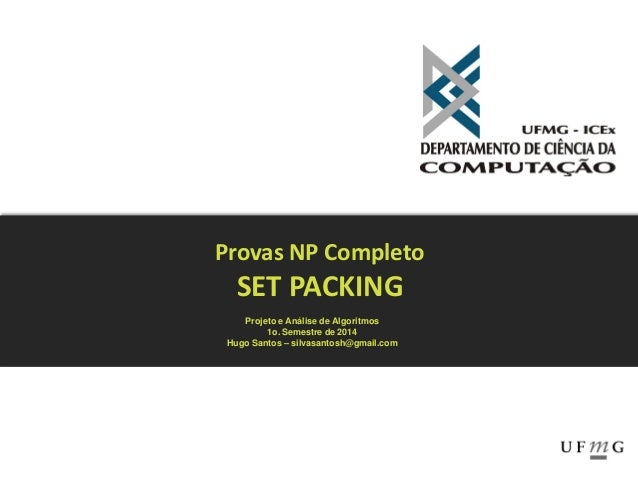Successfully reported this slideshow.
We use your LinkedIn profile and activity data to personalize ads and to show you more relevant ads. You can change your ad preferences anytime.Upcoming SlideShare
×

# Set packing - NP Proof / Prova NP Set Packing

472 views

Published on

Set packing is a classical NP-complete problem in computational complexity theory and combinatorics, and was one of Karp's 21 NP-complete problems.

Suppose we have a finite set S and a list of subsets of S. Then, the set packing problem asks if some k subsets in the list are pairwise disjoint (in other words, no two of them share an element).

Published in: Technology
• Full Name
Comment goes here.

Are you sure you want to Yes No
Your message goes here• Be the first to comment

• Be the first to like this

### Set packing - NP Proof / Prova NP Set Packing

1. 1. Provas NP Completo SET PACKING Projeto e Análise de Algoritmos 1o. Semestre de 2014 Hugo Santos – silvasantosh@gmail.com
2. 2. Definição O problema Set Packing pode ser definido como: Dado um universo U e uma família S dos subconjuntos de U, um packing é uma subfamília C ⊂ S dos conjuntos em que todos os conjuntos em C são disjuntos par-a- par. Na sua versão de problema de decisão a entrada é o par (U, S) e um inteiro k > 0. A pergunta a ser respondida é: existe um conjunto C, onde |C| ≥ k?
3. 3. Etapas da Prova NP-Completo 1) Mostrar que Set Packing (π) está em NP: a) problemas de decisão cuja solução pode ser verificada em tempo polinomial com algoritmo determinista. 2) Mostrar que um problema NP-completo conhecido (π‘) pode ser polinomialmente transformado para ele (π‘ α π).
4. 4. Mostrar que Set Packing (π) está em NP 1) Algoritmo determinista para verificar a solução em tempo polinomial: O primeiro passo é verificar se C possui no mínimo k elementos e na sequência verificar se cada par Si e Sj possui interseção vazia. A complexidade deste algoritmo pode ser dada por O(|C|2), onde m é o tamanho do maior conjunto pertencente a C. Logo é polinomial.
5. 5. Mostrar que um problema NP-completo conhecido (π‘) pode ser polinomialmente transformado para ele (π‘ α π) 1) Consideremos π‘ o problema dos conjuntos independentes (Independent Sets), que é sabidamente NP-Completo para seu problema de decisão. 2) Problema: Qual é o maior subconjunto de vertices de V tal que nenhum par de vertices são adjascentes?
6. 6. Mostrar que um problema NP-completo conhecido (π‘) pode ser polinomialmente transformado para ele (π‘ α π) 1) No problema de decisão do conjunto independente, a entrada é um grafo não direcionado e um número k, e a saída é um valor booleano: true se o gráfico contém um conjunto independente de tamanho k, e false caso contrário. 2) A intuição necessária é que cada vértice vi possui associado a ele a lista de arestas incidentes. A condição para que vi faca parte da solução é ele não possuir nenhuma aresta incidente comum aos demais vértices já presentes na solução. Então para transformar este problema em um Set Packing, basta criar um grafo G onde cada vértice vi possui um conjunto de arestas incidentes exatamente igual a cada Si existente em S e definir k’ = k.
7. 7. Mostrar que um problema NP-completo conhecido (π‘) pode ser polinomialmente transformado para ele (π‘ α π) 1) Para validar a solução, inicialmente iremos assumir que G possui um conjunto de vértices independentes com no mínimo k elementos, T. cada vi ϵ T iremos adicionar o conjunto de arestas incidentes, Si, em C. Note que |C| = |T| e que Si ∩ Sj = Ø; ∀Si,Sj ϵ C e i ≠ j, como |T| ≥ k podemos concluir que T é uma solução para o problema Set Packing.
8. 8. EXEMPLO: (π‘ α π)